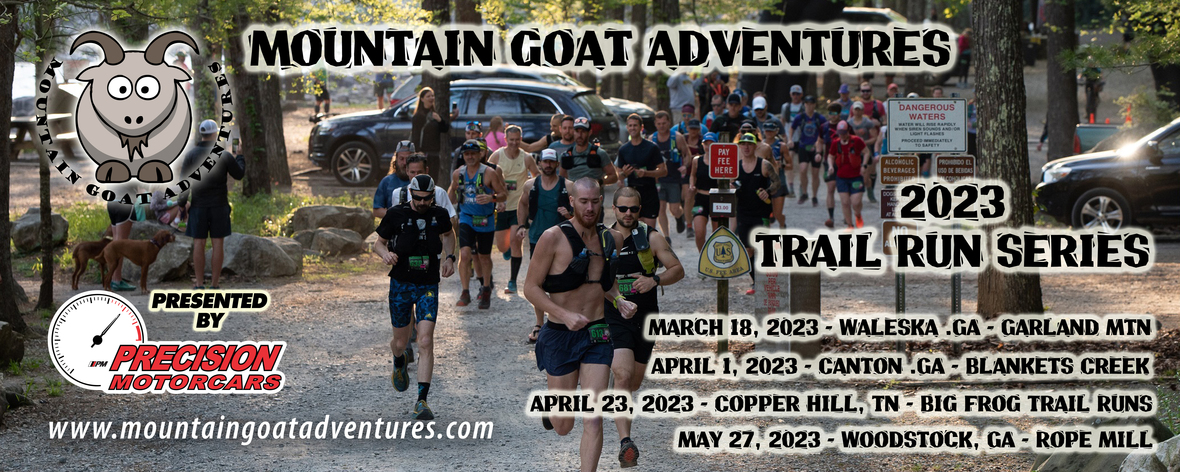# KEY DETAILS YOU NEED TO KNOW/ACTION ITEMS:

▪ RACE VENUE ADDRESS: Garland Mountain Horse and Hike Trails, 1411 Garland Mountain Way, Waleska, GA 30183
▪ Waivers need to be printed at home, filled out ahead of time, and brought to the race check-in CLICK HERE FOR EVENT WAIVER
▪ No race day distance changes - if you want to change distance, please contact me via email now and I'll get you taken care of. No changes after 3/13.
▪ If you can no longer attend, you may sell your entry to someone else, but this needs to be done by 3/13. Payment is between buyer and seller..all I need is the new runner's name and email no later than 3/13. No late transfers, and no transfers to other events at this point.
▪ SCHEDULE: 8 am - 13.1 mile start, 8:20 am - 10K start, 8:20 am
 ▪ RACE VENUE ADDRESS: Garland Mountain Horse and Hike Trails, 1411 Garland Mountain Way, Waleska, GA 30183
 ▪ Waivers need to be printed at home, filled out ahead of time, and brought to the race check-in CLICK HERE FOR EVENT WAIVER
 ▪ No race day distance changes - if you want to change distance, please contact me via email now and I'll get you taken care of. No changes after 3/13.
 ▪ If you can no longer attend, you may sell your entry to someone else, but this needs to be done by 3/13. Payment is between buyer and seller..all I need is the new runner's name and email no later than 3/13. No late transfers, and no transfers to other events at this point.
 ▪ SCHEDULE: 8 am - 13.1 mile start, 8:20 am - 10K start, 8:20 am
 table div table+table+table+table div table{width:100%;padding:0}table div table+table+table+table div table img{width:96.23%;padding:0;float:none}table div table+table+table+table div table td{width:100%;padding:0 1.88% 18px}/* styles */## CHECK-IN

▪ Saturday Check-in hours are from 6:30 am to 7:45 am. When you arrive, parking helpers will direct you where to park - please park where directed. Overflow parking is along the roadside on Garland Mountain Way. PLEASE NOTE THERE IS NOT AN EARLY WEEKNIGHT CHECK IN FOR THIS EVENT - ALL PACKET PICKUP IS ON SATURDAY MORNING.
▪ Proceed to appropriate check-in line with waiver. Hand sanitizer will be available.
▪ Port-o-lets and a hand wash station will be available at this venue.
▪ If you registered for the entire Trail Run Series, you will receive your hooded sweatshirt at check in before the race. We have 6 colors available (shown below) so please come early if you have your heart set on a particular color. Sweatshirts will be available for purchase to non-series registrants AFTER the race for \$25 each.
 ▪ Saturday Check-in hours are from 6:30 am to 7:45 am. When you arrive, parking helpers will direct you where to park - please park where directed. Overflow parking is along the roadside on Garland Mountain Way. PLEASE NOTE THERE IS NOT AN EARLY WEEKNIGHT CHECK IN FOR THIS EVENT - ALL PACKET PICKUP IS ON SATURDAY MORNING.
 ▪ Proceed to appropriate check-in line with waiver. Hand sanitizer will be available.
 ▪ Port-o-lets and a hand wash station will be available at this venue.
 ▪ If you registered for the entire Trail Run Series, you will receive your hooded sweatshirt at check in before the race. We have 6 colors available (shown below) so please come early if you have your heart set on a particular color. Sweatshirts will be available for purchase to non-series registrants AFTER the race for \$25 each.

## RACE PROCEDURES

▪ Make sure your number is pinned on your front - do not bend the foam timing chip. Number belts are fine, just make sure it is on your front as you pass over the timing mats. Your time starts when you cross over the timing mats.
▪ Brief pre-race meeting at 7:45 am in front of the bathrooms.
▪ Water station at Mile 4 and 5.5 on the 10k course, and Mile 4, 7.9, 9.2 and 12.5 on the 13.1 course. Water, Hammer Heed and Hammer Gels will be available. No throwing cups or trash on the ground. Hand sanitizer will be available at the aid station. Do not throw trash anywhere on the course - penalty is DQ.
▪ Course will be well marked with pink ribbons and pink/black arrow signs at turns. Pink ribbons indicate the direction you want to head and are also used to designate that you are on-course if it has been a while in between turns. Course marshals will be positioned throughout the course for your safety.
▪ ANNOUNCE if you are passing to runners ahead and say PASSING RIGHT or PASSING LEFT and give them a chance to move to the side. PLEASE BE PATIENT, WORK TOGETHER, BE NICE and give each other space out on the trails.
 ▪ Make sure your number is pinned on your front - do not bend the foam timing chip. Number belts are fine, just make sure it is on your front as you pass over the timing mats. Your time starts when you cross over the timing mats.
 ▪ Brief pre-race meeting at 7:45 am in front of the bathrooms.
 ▪ Water station at Mile 4 and 5.5 on the 10k course, and Mile 4, 7.9, 9.2 and 12.5 on the 13.1 course. Water, Hammer Heed and Hammer Gels will be available. No throwing cups or trash on the ground. Hand sanitizer will be available at the aid station. Do not throw trash anywhere on the course - penalty is DQ.
 ▪ Course will be well marked with pink ribbons and pink/black arrow signs at turns. Pink ribbons indicate the direction you want to head and are also used to designate that you are on-course if it has been a while in between turns. Course marshals will be positioned throughout the course for your safety.
 ▪ ANNOUNCE if you are passing to runners ahead and say PASSING RIGHT or PASSING LEFT and give them a chance to move to the side. PLEASE BE PATIENT, WORK TOGETHER, BE NICE and give each other space out on the trails.

## RESULTS/AWARDS

▪ Finish times wlll be uploaded to our results website, mgaresults.com , so you can view them on your mobile device or you can view them on tablets at our results kiosk.
▪ 10k awards at approximately 10 am and 13.1 mile awards at 11 am. Series awards to follow each award ceremeony.
AWARDS WILL NOT BE MAILED - THEY MUST BE PICKED UP AT THE RACE
 ▪ Finish times wlll be uploaded to our results website, mgaresults.com , so you can view them on your mobile device or you can view them on tablets at our results kiosk.
 ▪ 10k awards at approximately 10 am and 13.1 mile awards at 11 am. Series awards to follow each award ceremeony. AWARDS WILL NOT BE MAILED - THEY MUST BE PICKED UP AT THE RACE
 table div table+table+table+table+table+table+table div table{width:100%;padding:0}table div table+table+table+table+table+table+table div table img{width:96.23%;padding:0;float:none}table div table+table+table+table+table+table+table div table td{width:100%;padding:0 1.88% 18px}/* styles */## New Mountian Goat Hoodies and Hats Available at this Event!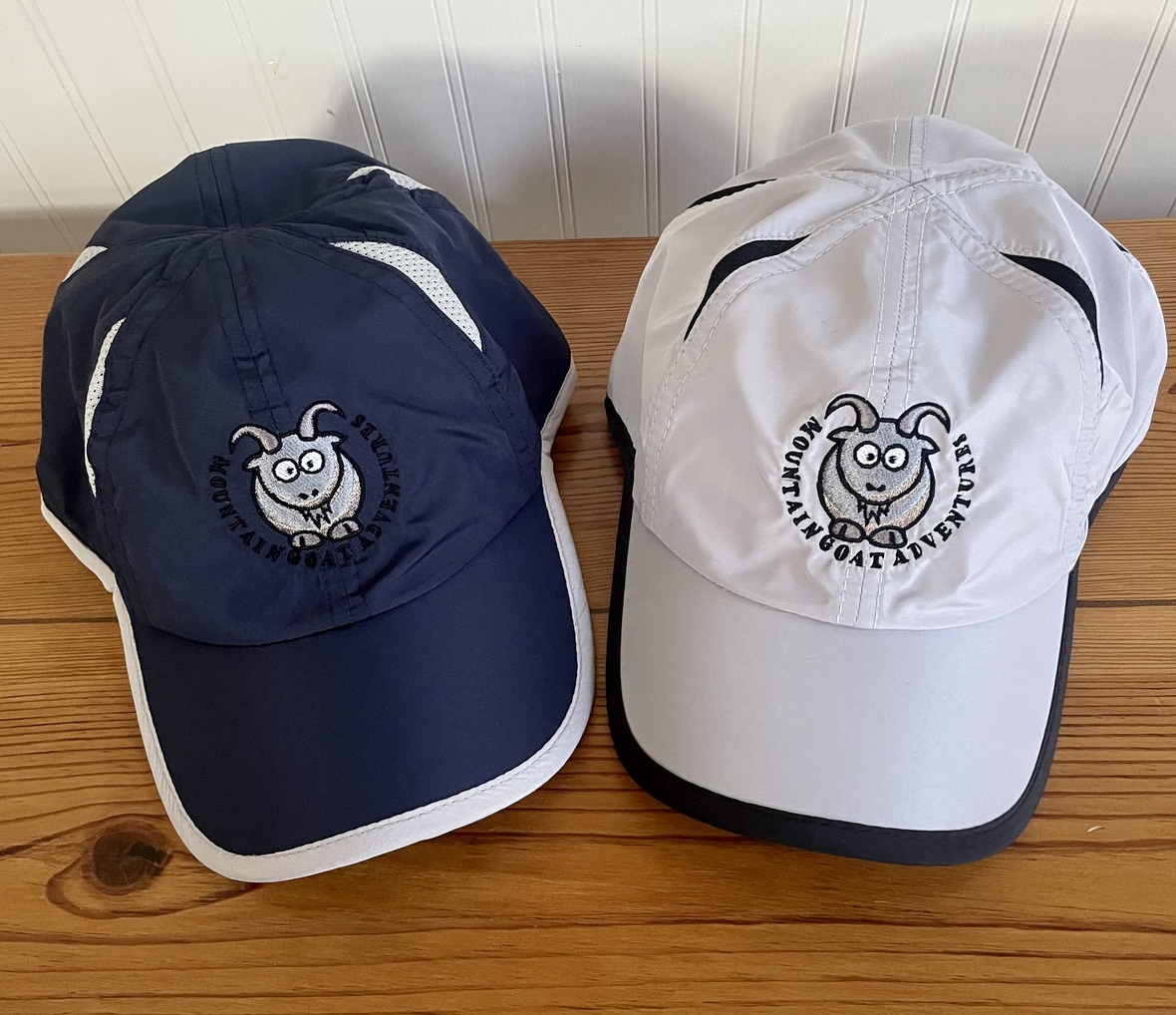If you weren't able to sign up for the entire Series but would like our newest Mountain Goat hoody, we will have them available for purchase for \$25. These are available in a variety of colors in sizes S - XXL. Cash or Venmo.

We also have new dry fit running hats for \$15 as well as wool blend trucker caps for \$20.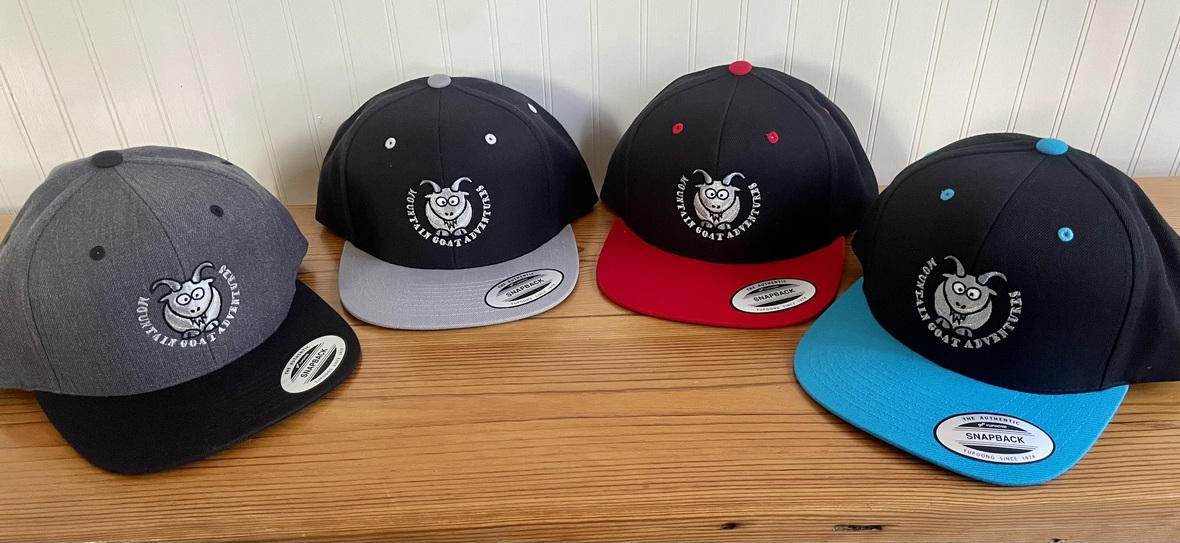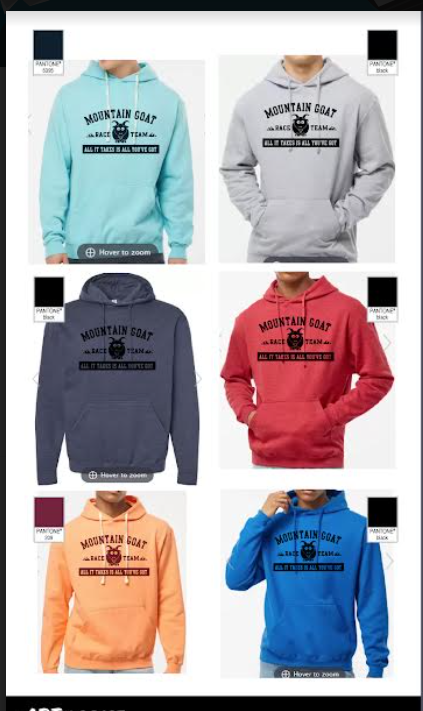2023 Sweatshirt Colors
 table div table+table+table+table+table+table+table+table+table+table+table+table div table{width:100%;padding:0}table div table+table+table+table+table+table+table+table+table+table+table+table div table img{width:96.23%;padding:0;float:none}table div table+table+table+table+table+table+table+table+table+table+table+table div table td{width:100%;padding:0 1.88% 18px}/* styles */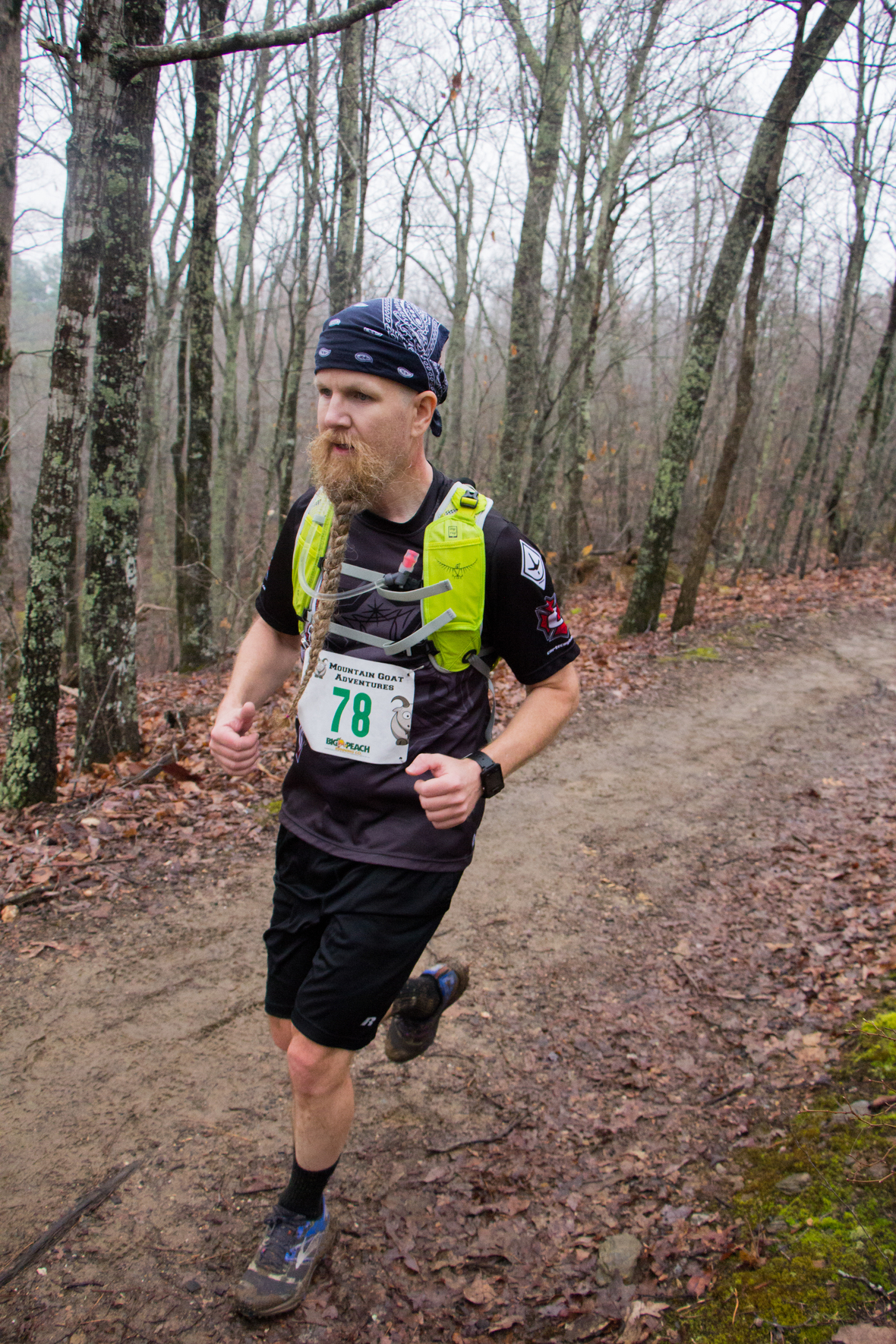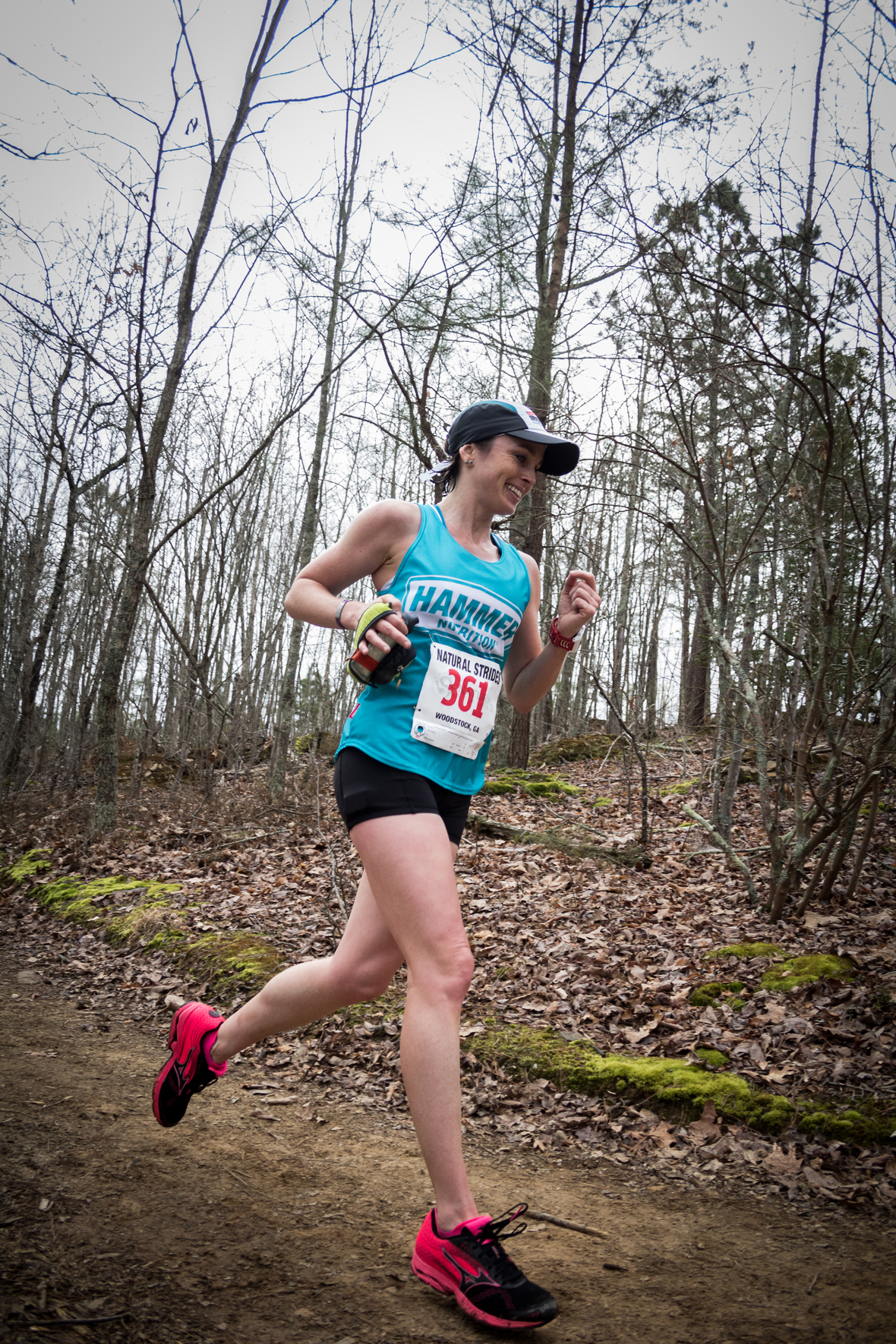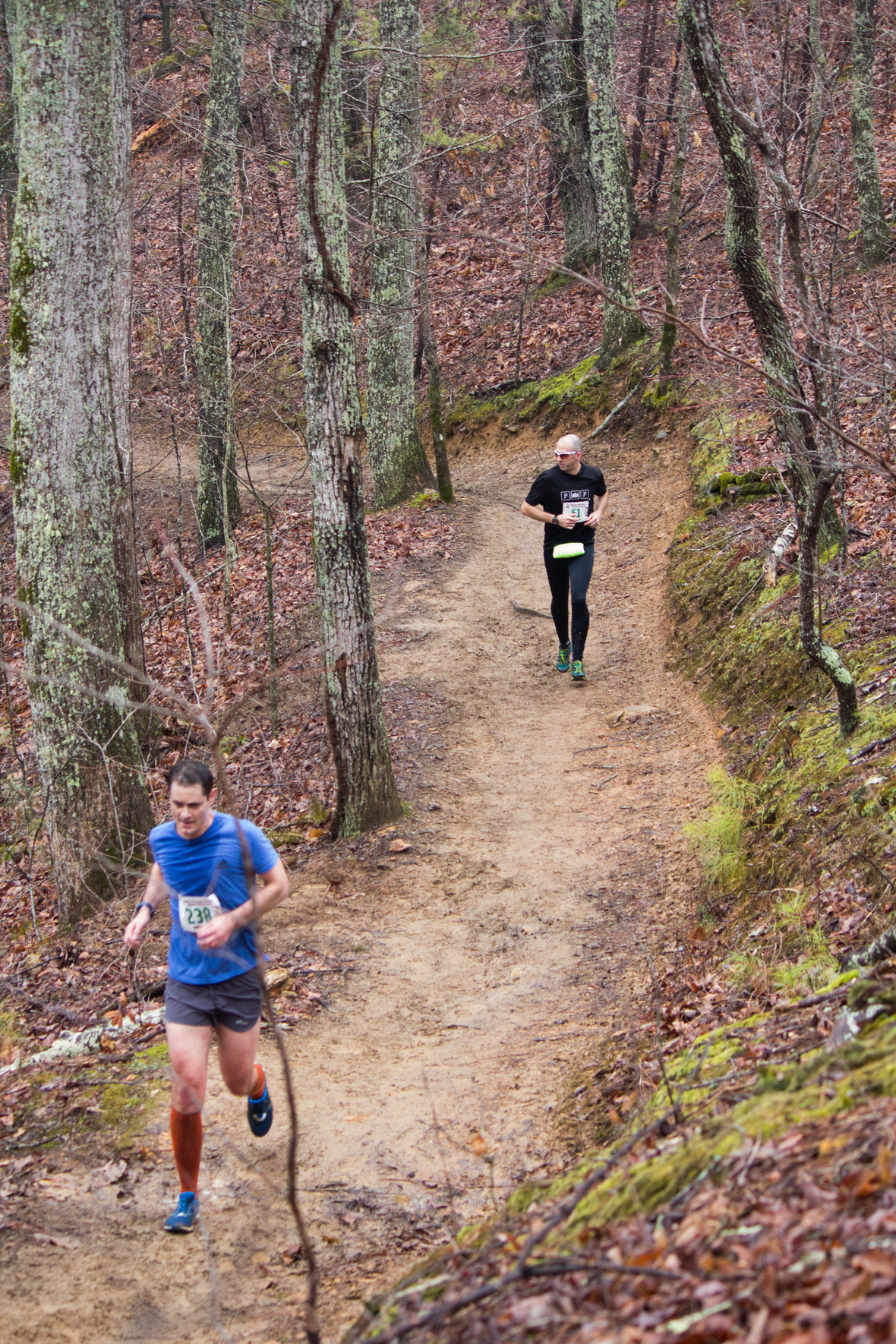table div table+table+table+table+table+table+table+table+table+table+table+table+table+table div table{width:100%;padding:0}table div table+table+table+table+table+table+table+table+table+table+table+table+table+table div table img{width:96.23%;padding:0;float:none}table div table+table+table+table+table+table+table+table+table+table+table+table+table+table div table td{width:100%;padding:0 1.88% 18px}/* styles */## Fueling Tips from Hammer Nutrition

 table div table+table+table+table+table+table+table+table+table+table+table+table+table+table+table+table div table,table.module-15{width:50.75%;float:right;padding:0}table div table+table+table+table+table+table+table+table+table+table+table+table+table+table+table+table div table a{border:0 none;text-decoration:none}table div table+table+table+table+table+table+table+table+table+table+table+table+table+table+table+table div table img{width:100%!important;border:0 none;text-decoration:none}table div table+table+table+table+table+table+table+table+table+table+table+table+table+table+table+table div table td{width:100%;padding:0 0 20px 20px}/* styles */ Your body needs to run at peak capacity so you can get your best result during the race. Here are a few tips from Hammer Nutrition to get started and make sure your body runs at peak capacity from start to finish! Tip #1 Hydration: Replenish, don’t replace! Keep fluid intake below 25 oz./hr during exercise. FIND OUT HOW MUCH Tip #2 Caloric Intake: Stay fueled, but not overwhelmed, by consuming 120-180 calories/hr. ARE CARBS BEST? Tip #3 Electrolytes: You need an array of vitamins and minerals, not just salt, to finish strong. WHAT DO YOU NEED? Tip #4 Pre-Event Fueling: Don’t tax your system with a meal within 3 hours of start time. BUT WHAT IF YOU'RE HUNGRY? Tip #5 Recovery: Refuel your tank with 60 minutes of finishing a workout. ARE CARBS OR PROTEIN BETTER?
 table div table+table+table+table+table+table+table+table+table+table+table+table+table+table+table+table+table div table{width:100%;padding:0}table div table+table+table+table+table+table+table+table+table+table+table+table+table+table+table+table+table div table img{width:96.23%;padding:0;float:none}table div table+table+table+table+table+table+table+table+table+table+table+table+table+table+table+table+table div table td{width:100%;padding:0 1.88% 18px}/* styles */## Visit Mulberry Gap Adventure Basecamp in Ellijay for Mountain Running and Amazing Meals!

 table div table+table+table+table+table+table+table+table+table+table+table+table+table+table+table+table+table+table+table div table td,table.module-18{width:100%;padding:0}table div table+table+table+table+table+table+table+table+table+table+table+table+table+table+table+table+table+table+table div table{width:100%;float:none;margin-left:auto;margin-right:auto;padding:0}table div table+table+table+table+table+table+table+table+table+table+table+table+table+table+table+table+table+table+table div table a{border:0 none;text-decoration:none}table div table+table+table+table+table+table+table+table+table+table+table+table+table+table+table+table+table+table+table div table img{width:100%!important;border:0 none;text-decoration:none}/* styles */
 table div table+table+table+table+table+table+table+table+table+table+table+table+table+table+table+table+table+table+table+table div table td,table.module-19{width:100%;padding:0}table div table+table+table+table+table+table+table+table+table+table+table+table+table+table+table+table+table+table+table+table div table{width:100%;float:none;margin-left:auto;margin-right:auto;padding:0}table div table+table+table+table+table+table+table+table+table+table+table+table+table+table+table+table+table+table+table+table div table a{border:0 none;text-decoration:none}table div table+table+table+table+table+table+table+table+table+table+table+table+table+table+table+table+table+table+table+table div table img{width:100%!important;border:0 none;text-decoration:none}/* styles */
 table div table+table+table+table+table+table+table+table+table+table+table+table+table+table+table+table+table+table+table+table+table div table{width:100%;padding:0}table div table+table+table+table+table+table+table+table+table+table+table+table+table+table+table+table+table+table+table+table+table div table img{width:96.23%;padding:0;float:none}table div table+table+table+table+table+table+table+table+table+table+table+table+table+table+table+table+table+table+table+table+table div table td{width:100%;padding:0 1.88% 18px}/* styles */## 2023 Full Event Calendar

 /* styles */ Want to check out our other events? Our 2023 calendar is below - we focus on mountain bike races, trail runs, and off-road duathlons. March 18, 2023 - Assault on Garland Mountain 10k and 13.1 mile Trail Run April 1, 2023 - Blankets Creek Dirty 15/30 Mountain Bike Race April 1, 2023 - Blankets Creek 5 and 10 mile Trail Run April 23, 2023 - Big Frog 50k - 13.1 mile - 7 mile Trail Runs May 27, 2023 - Rope Mill 10k and 13.1 mile Trail Run May 27, 2023 - Rope Mill Fast+Furious 15 MTB Race September 9, 2023 - Fools Gold 38 and 60 Mile MTB Races September 30, 2023 - Blankets Creek 3/6 Hour Race November 11, 2023 - Rope Mill Dirty Duathlon and 5k Trail Run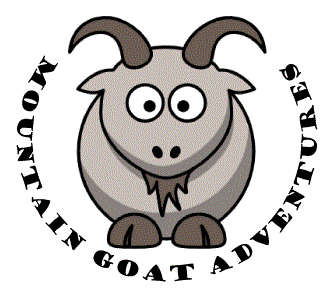## Thanks to our Trail Run Sponsors!

 table div table+table+table+table+table+table+table+table+table+table+table+table+table+table+table+table+table+table+table+table+table+table+table+table+table+table div table td,table.module-25{width:100%;padding:0}table div table+table+table+table+table+table+table+table+table+table+table+table+table+table+table+table+table+table+table+table+table+table+table+table+table+table div table{width:100%;float:none;margin-left:auto;margin-right:auto;padding:0}table div table+table+table+table+table+table+table+table+table+table+table+table+table+table+table+table+table+table+table+table+table+table+table+table+table+table div table a{border:0 none;text-decoration:none}table div table+table+table+table+table+table+table+table+table+table+table+table+table+table+table+table+table+table+table+table+table+table+table+table+table+table div table img{width:100%!important;border:0 none;text-decoration:none}/* styles */
 table div table+table+table+table+table+table+table+table+table+table+table+table+table+table+table+table+table+table+table+table+table+table+table+table+table+table+table div table{width:100%;padding:0}table div table+table+table+table+table+table+table+table+table+table+table+table+table+table+table+table+table+table+table+table+table+table+table+table+table+table+table div table table{padding:0;float:left!important;width:61.509%!important}table div table+table+table+table+table+table+table+table+table+table+table+table+table+table+table+table+table+table+table+table+table+table+table+table+table+table+table div table table+table td,table div table+table+table+table+table+table+table+table+table+table+table+table+table+table+table+table+table+table+table+table+table+table+table+table+table+table+table div table td{padding-left:0;padding-right:0}table div table+table+table+table+table+table+table+table+table+table+table+table+table+table+table+table+table+table+table+table+table+table+table+table+table+table+table div table table td{padding-left:0;padding-right:20px}table div table+table+table+table+table+table+table+table+table+table+table+table+table+table+table+table+table+table+table+table+table+table+table+table+table+table+table div table table+table{float:left!important;width:38.491%!important}/* styles */
 table div table+table+table+table+table+table+table+table+table+table+table+table+table+table+table+table+table+table+table+table+table+table+table+table+table+table+table+table div table{width:100%;padding:0}table div table+table+table+table+table+table+table+table+table+table+table+table+table+table+table+table+table+table+table+table+table+table+table+table+table+table+table+table div table table{padding:0;float:left!important;width:61.32%!important}table div table+table+table+table+table+table+table+table+table+table+table+table+table+table+table+table+table+table+table+table+table+table+table+table+table+table+table+table div table table+table td,table div table+table+table+table+table+table+table+table+table+table+table+table+table+table+table+table+table+table+table+table+table+table+table+table+table+table+table+table div table td{padding-left:0;padding-right:0}table div table+table+table+table+table+table+table+table+table+table+table+table+table+table+table+table+table+table+table+table+table+table+table+table+table+table+table+table div table table td{padding-left:0;padding-right:20px}table div table+table+table+table+table+table+table+table+table+table+table+table+table+table+table+table+table+table+table+table+table+table+table+table+table+table+table+table div table table+table{float:left!important;width:38.68%!important}/* styles */
 table div table+table+table+table+table+table+table+table+table+table+table+table+table+table+table+table+table+table+table+table+table+table+table+table+table+table+table+table+table div table{width:100%;padding:0}table div table+table+table+table+table+table+table+table+table+table+table+table+table+table+table+table+table+table+table+table+table+table+table+table+table+table+table+table+table div table table{padding:0;float:left!important;width:56.415%!important}table div table+table+table+table+table+table+table+table+table+table+table+table+table+table+table+table+table+table+table+table+table+table+table+table+table+table+table+table+table div table table+table td,table div table+table+table+table+table+table+table+table+table+table+table+table+table+table+table+table+table+table+table+table+table+table+table+table+table+table+table+table+table div table td{padding-left:0;padding-right:0}table div table+table+table+table+table+table+table+table+table+table+table+table+table+table+table+table+table+table+table+table+table+table+table+table+table+table+table+table+table div table table td{padding-left:0;padding-right:20px}table div table+table+table+table+table+table+table+table+table+table+table+table+table+table+table+table+table+table+table+table+table+table+table+table+table+table+table+table+table div table table+table{float:left!important;width:43.585%!important}/* styles */
 table div table+table+table+table+table+table+table+table+table+table+table+table+table+table+table+table+table+table+table+table+table+table+table+table+table+table+table+table+table+table div table{width:100%;padding:0}table div table+table+table+table+table+table+table+table+table+table+table+table+table+table+table+table+table+table+table+table+table+table+table+table+table+table+table+table+table+table div table table{padding:0;float:left!important;width:41.886%!important}table div table+table+table+table+table+table+table+table+table+table+table+table+table+table+table+table+table+table+table+table+table+table+table+table+table+table+table+table+table+table div table table+table td,table div table+table+table+table+table+table+table+table+table+table+table+table+table+table+table+table+table+table+table+table+table+table+table+table+table+table+table+table+table+table div table td{padding-left:0;padding-right:0}table div table+table+table+table+table+table+table+table+table+table+table+table+table+table+table+table+table+table+table+table+table+table+table+table+table+table+table+table+table+table div table table td{padding-left:0;padding-right:20px}table div table+table+table+table+table+table+table+table+table+table+table+table+table+table+table+table+table+table+table+table+table+table+table+table+table+table+table+table+table+table div table table+table{float:left!important;width:58.114%!important}/* styles */
 table.module-30{width:55.85%;padding:0}table div table+table+table+table+table+table+table+table+table+table+table+table+table+table+table+table+table+table+table+table+table+table+table+table+table+table+table+table+table+table+table div table{width:55.85%;float:none;margin-left:auto;margin-right:auto;padding:0}table div table+table+table+table+table+table+table+table+table+table+table+table+table+table+table+table+table+table+table+table+table+table+table+table+table+table+table+table+table+table+table div table a{border:0 none;text-decoration:none}table div table+table+table+table+table+table+table+table+table+table+table+table+table+table+table+table+table+table+table+table+table+table+table+table+table+table+table+table+table+table+table div table img{width:100%!important;border:0 none;text-decoration:none}table div table+table+table+table+table+table+table+table+table+table+table+table+table+table+table+table+table+table+table+table+table+table+table+table+table+table+table+table+table+table+table div table td{width:100%;padding:0}/* styles */